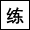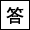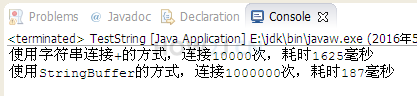数字与字符串系列教材 （八）- Java 比较字符串详解 数字与字符串系列教材 （十）- 自己开发一个Java StringBuffer

StringBuffer是可变长的字符串5分9秒

append delete insert reverse

length capacity## 追加 删除 插入 反转

append追加
delete 删除
insert 插入
reverse 反转
package character; public class TestString { public static void main(String[] args) { String str1 = "let there "; StringBuffer sb = new StringBuffer(str1); //根据str1创建一个StringBuffer对象 sb.append("be light"); //在最后追加 System.out.println(sb); sb.delete(4, 10);//删除4-10之间的字符 System.out.println(sb); sb.insert(4, "there ");//在4这个位置插入 there System.out.println(sb); sb.reverse(); //反转 System.out.println(sb); } }

## 长度 容量

length: “the”的长度 3
capacity: 分配的总空间 19

package character; public class TestString { public static void main(String[] args) { String str1 = "the"; StringBuffer sb = new StringBuffer(str1); System.out.println(sb.length()); //内容长度 System.out.println(sb.capacity());//总空间 } }
```package character;

public class TestString {

public static void main(String[] args) {
String str1 = "the";

StringBuffer sb = new StringBuffer(str1);

System.out.println(sb.length()); //内容长度

System.out.println(sb.capacity());//总空间

}

}

```

## 练习-StringBuffer性能

姿势不对,事倍功半! 点击查看做练习的正确姿势
String与StringBuffer的性能区别?## 答案-StringBuffer性能7分27秒 本视频采用html5方式播放，如无法正常播放，请将浏览器升级至最新版本，推荐火狐，chrome，360浏览器。 如果装有迅雷，播放视频呈现直接下载状态，请调整 迅雷系统设置-基本设置-启动-监视全部浏览器 (去掉这个选项)。 chrome 的 视频下载插件会影响播放，如 IDM 等，请关闭或者切换其他浏览器

package character; public class TestString { public static void main(String[] args) { int total = 10000; String s = randomString(10); StringBuffer sb = new StringBuffer(); String str1 = ""; long start = System.currentTimeMillis(); for (int i = 0; i <total; i++) { str1+=s; } long end = System.currentTimeMillis(); System.out.printf("使用字符串连接+的方式，连接%d次，耗时%d毫秒%n",total,end-start); total *=100; start = System.currentTimeMillis(); for (int i = 0; i <total; i++) { sb.append(s); } end = System.currentTimeMillis(); System.out.printf("使用StringBuffer的方式，连接%d次，耗时%d毫秒%n",total,end-start); } private static String randomString(int length) { String pool = ""; for (short i = '0'; i <= '9'; i++) { pool += (char) i; } for (short i = 'a'; i <= 'z'; i++) { pool += (char) i; } for (short i = 'A'; i <= 'Z'; i++) { pool += (char) i; } char cs[] = new char[length]; for (int i = 0; i < cs.length; i++) { int index = (int) (Math.random() * pool.length()); cs[i] = pool.charAt(index); } String result = new String(cs); return result; } }

## 练习-MyStringBuffer

姿势不对,事倍功半! 点击查看做练习的正确姿势

package character; public interface IStringBuffer { public void append(String str); //追加字符串 public void append(char c); //追加字符 public void insert(int pos,char b); //指定位置插入字符 public void insert(int pos,String b); //指定位置插入字符串 public void delete(int start); //从开始位置删除剩下的 public void delete(int start,int end); //从开始位置删除结束位置-1 public void reverse(); //反转 public int length(); //返回长度 }
```package character;

public interface IStringBuffer {
public void append(String str); //追加字符串
public void append(char c);  //追加字符
public void insert(int pos,char b); //指定位置插入字符
public void insert(int pos,String b); //指定位置插入字符串
public void delete(int start); //从开始位置删除剩下的
public void delete(int start,int end); //从开始位置删除结束位置-1
public void reverse(); //反转
public int length(); //返回长度
}
```
package character; public class MyStringBuffer implements IStringBuffer{ }
```package character;
public class MyStringBuffer implements IStringBuffer{
}
```

## 答案-MyStringBuffer

 数字与字符串系列教材 （八）- Java 比较字符串详解 数字与字符串系列教材 （十）- 自己开发一个Java StringBuffer

HOW2J公众号，关注后实时获知最新的教程和优惠活动，谢谢。2022-05-27 答案
 芬达味橘猫 答案```package newpkg1; public class MyStringBuffer implements IStringBuffer{ String str; public MyStringBuffer(String str){ this.str = str; } @Override public void append(String str) { this.str += str; } @Override public void append(char c) { this.str += c; } @Override public void insert(int pos, char b) { int sl = this.str.length(); String head = this.str.substring(0,pos+1); String tail = this.str.substring(pos+1,sl); this.str = head + b + tail; } @Override public void insert(int pos, String b) { int sl = this.str.length(); String head = this.str.substring(0,pos+1); String tail = this.str.substring(pos+1,sl); this.str = head + b + tail; } @Override public void delete(int start) { this.str = this.str.substring(0,start); } @Override public void delete(int start, int end) { int sl = this.str.length(); String head = this.str.substring(0,start+1); String tail = this.str.substring(end,sl); this.str = head + tail; } @Override public void reverse() { int sl = this.str.length(); char[] temp = this.str.toCharArray(); this.str = ""; for (int i = sl - 1;i >= 0;i--){ this.str += temp[i]; } } @Override public int length() { return this.str.length(); } @Override public String toString() { return this.str; } public static void main(String[] args) { String tomas = "快给白傻子买瓜子"; char cs = '去'; String ss = "去！！"; MyStringBuffer tsb = new MyStringBuffer(tomas); //初始字符串 System.out.println(tsb); //添加 tsb.append(cs); System.out.println(tsb); tsb.append(ss); System.out.println(tsb); //插入 cs = '儿'; tsb.insert(7,cs); System.out.println(tsb); ss = "一斤"; tsb.insert(5,ss); System.out.println(tsb); //删除 tsb.delete(11); System.out.println(tsb); tsb.delete(5,8); System.out.println(tsb); //翻转 tsb.reverse(); System.out.println(tsb); //长度 System.out.println("字符长度为"+tsb.length()+"个"); } } ```

2022-05-10 练习1
 大D 不确定对不对 ```public static void main(String[] args) { String str1 = "let there "; StringBuffer sb =new StringBuffer(str1); sb.delete(4,10); sb.insert(4,"aaa"); sb.reverse(); System.out.println(sb+""+sb.length()+sb.capacity()); String zfc=random(10) ; System.out.println(zfc); long startTime=System.currentTimeMillis(); for(int i=0;i<10000;i++){ zfc+=random(10); } long endTime=System.currentTimeMillis(); System.out.println(endTime-startTime); StringBuffer sf=new StringBuffer(zfc); long startTime2=System.currentTimeMillis(); for(int i=0;i<10000;i++){ sf.append(random(10)); } long endTime2=System.currentTimeMillis(); System.out.println(endTime2-startTime2); } public static String random(int length){ String result =""; for(short i=0;i<=9;i++){ result+=(char)i; } for(short i='a';i<='z';i++){ result+=(char)i; } for(short i='A';i<='Z';i++){ result+=(char)i; } char[] chars =new char[length]; for(int i=0;i

1 个答案

import java.util.Random; public class s7 { public static void main(String[] args) { long sstart = System.currentTimeMillis(); Random rand = new Random(); String letter = "abcdefghijklmnopqrstuvwxyzABCDEFGHIJKLMNOPQRSTUVWXYZ0123456789"; String tomas = ""; String str; for (int i = 0;i<10000;i++) { str = String.valueOf(letter.charAt(rand.nextInt(letter.length()))); tomas += str; } long ssend = System.currentTimeMillis(); long ssys = ssend - sstart; System.out.println("使用字符串连接，连接10000次，耗时"+ssys+"毫秒"); String wang = ""; long sbstart = System.currentTimeMillis(); StringBuffer sb = new StringBuffer(wang); for (int i = 0;i<10000;i++) { sb.append(letter.charAt(rand.nextInt(letter.length()))); } long sbend = System.currentTimeMillis(); long sbys = sbend - sbstart; System.out.println("使用StringBuffer连接，连接10000次，耗时"+sbys+"毫秒"); } }

2022-03-03 我自己的答案，很笨的答案，不过挺容易懂的。
2021-06-15 答案
2021-05-14 练习MyStringBuffer